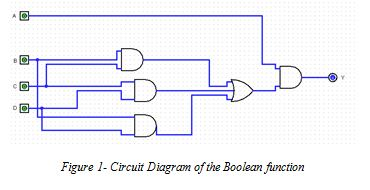# ITC544 Data Representation and Digital Logic Editing Services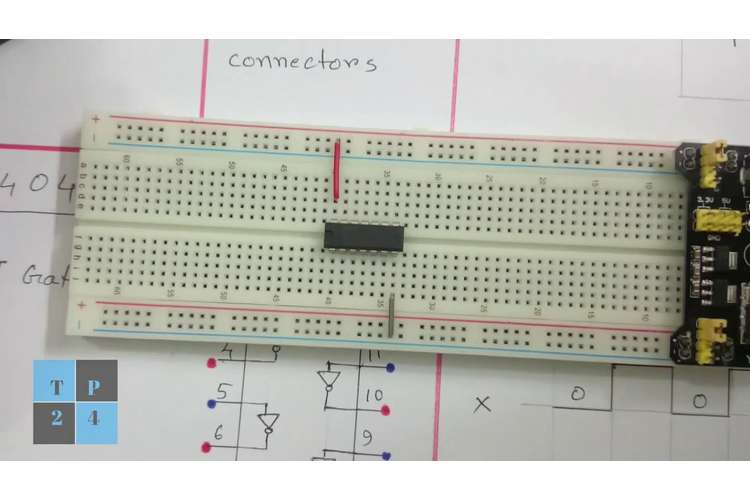## Question 1

### Part (a)

As the word size is given as 8 bits, therefore, n=8 can be taken.

(I) For One’s complement

The general representation range of 1’s complement is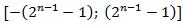Therefore, the range of 1’s complement is going to be: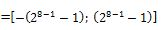= [-127; 127]

After that converting them into binary I get,

10000000 to 11111111 which represents -127 to -0.

(II) For two’s complement

The general representation range of the 2’s complement is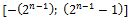Therefore, the range of 2’s complement is going to be: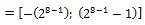= [-128; 127]

Now on converting the range into the binary equivalent I get,

10000000 to 11111111 which is going to represent -128 to -1.

(III) For signed magnitude

The general representation range is -2(n-1) to +2(n-1)

On substituting the n=8 I get,

= -2(8-1) to +2(8-1)

= -128 to 128

On converting the rage into decimal equivalent I get,

00000000 to 01111111 which is going to represent 0 to 127.

(III) For the unsigned magnitude

The range is going to be 10000000 to 11111111.

It is going to represent 128 to 255.

### Part (b)

I. 0x5AB into Octal

As 0x represents the Hexadecimal number. So the conversion of the hexadecimal to the octal number is going to be as follow:

Firstly, I am going to convert the hexadecimal number to the decimal equivalent. For that certain equation is required which is shown below:

= 5*162 +10*161 +11*160

=1280+160+11

= (1451)10

Now to convert into the octal certain procedures has to be followed which is shown below: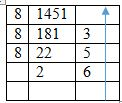So the octal number is (2653)8or it can be represented as 0o2653.

II. 101101.1012 into Decimal

For that I have to follow certain steps which is shown below:

=1*25+ 0*24+ 1*22+ 0*21+ 1*20

= 32 + 0 + 8 + 4 + 0 + 1

= 45

Now, for decimal part i.e. 101,

I have done certain calculation which is shown below:

= 1*2-1+ 0*2-2+1*2-3

= 0.5 + 0 + 0.125

= 0.625

On combining both part, solution is going to be:

= (45.625)10

III. 12348 into Binary

To perform this conversion, I have to convert this into decimal. For that certain steps have to be followed which are shown below:

= 1*83+ 2*82+ 3*81+ 4*80

= 512+ 128 + 24 + 4

= (668)10

Now I need to convert into the binary equivalent which is shown below: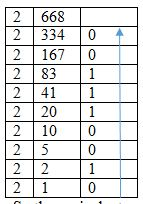So the equivalent number is going to be (1010011100)2

IV. 679810into Base 5 number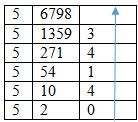So the equivalent number is going to be (204143)5

V. 976.6310 into Binary

Firstly, converting 976 into the base 10,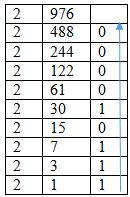Now converting .63 into the binary equivalent. For that certain procedure has to be followed which is shown below:

=0.63*2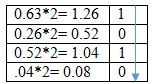On combining,

(1111010000.101)2

By taking the help of the table which is shown below: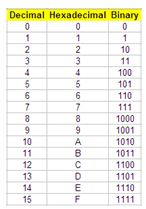Conversion is going to be implemented by grouping four numbers starting from right side to the left side. After by taking the help of table I get,

0010- 2

0100- 4

1011- B

So the desired result is going to be 24B.

VII. 10011110 (8-bit 2’s complement representation) to Decimal

On solving unsigned:

=12? + 02? + 02? + 12? + 12³ + 12² + 12¹ + 02?

=12? + 12? + 12³ + 12² + 1

=128 + 16 + 8 + 4 + 2

=158

On solving signed magnitude which is (bit 12? means negative value)

= 02? + 02? + 12? + 12³ + 12² + 12¹ + 02?

=12? + 12³ + 12² + 1

=16 + 8 + 4 + 2

= -30 (on applying the sign)

On solving one's complement which is (bit 12? means negative value, therefore I have to complement all bits)

Step 1 = (12? + 02? + 02? + 12? + 12³ + 12² + 12¹ + 02?)

Step 2 =02? + 12? + 12? + 02? + 02³ + 02² + 02¹ + 12?

=12? + 12? + 12?

=64 + 32 + 1

= -97 (on applying the sign)

On solving Two's complement which is (bit 12? means negative, complement bits, add 1)

= (12? + 02? + 02? + 12? + 12³ + 12² + 12¹ + 02?) + 1

= (02? + 12? + 12? + 02? + 02³ + 02² + 02¹ + 12?) + 1

= (12? + 12? + 12?) + 1

= (64 + 32 + 1) + 1

= -98 (on applying the sign)

### Question 2

#### Part (a)

According to question X’(X+Y) + (XX+Y) (Y’+X) = Y+X I need to prove this.

Taking LHS I get,

=X’(X+Y) + (XX+Y) (Y’+X)

On expanding I get,

=X’X+ X’Y+(X+Y) (Y’+X)

Since, X’X=0 therefore,

=0+X’Y+XY’+XX+YY’+YX

=0+X’Y+XY’+X+0+YX

Taking X common I get,

=X’Y + X(1+Y’) + YX

And 1+Y’=1

Therefore, the above expression becomes,

=X’Y+X+XY

The expression becomes,

=X’Y+X.X+XY+XX’

Taking X and Y out, the expression is going to as shown below:

=X(X’+X) + Y(X+X’)

=(X+Y) (X+X’)

Since X+X’=1

Therefore, on substituting inside the expression, it becomes,

=(X+Y) (1)

X+Y=RHS

#### Part (b)

As the subject has 4 assessments, therefore the four inputs are required. I have taken four inputs which are shown below:

A. Assignment

B. Blog

C. Discussion form

D. Quiz

As the student is going to be pass only if he passes two or more than two assessments.

So the function is going to be BC+CD+BD

Also, the student has to pass the assignment with 50 or above. And on analysing the problem, I have taken output as Y.

So the function of the above problem is going to be:

Y=A.(BC+CD+BD)

The truth table of the above circuit is shown below:

 A B C D Y 0 0 0 0 0 0 0 0 1 0 0 0 1 0 0 0 0 1 1 0 0 1 0 0 0 0 1 0 1 0 0 1 1 0 0 0 1 1 1 0 1 0 0 0 0 1 0 0 1 0 1 0 1 0 0 1 0 1 1 1 1 1 0 0 0 1 1 0 1 1 1 1 1 0 1 1 1 1 1 1

The minimized Boolean function can be built by taking the help of K-Map. This is shown below: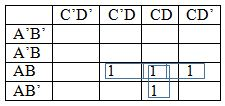And the minimised expression is going to be:

=ACD + ABD + ABC